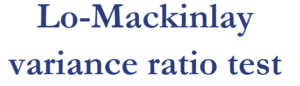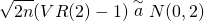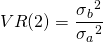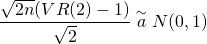# Variance ratio test

The Lo-Mackinlay variance ratio test of a random walk is a test used to determine whether securities indeed follow a random walk. The variance ratio (VR) test was proposed by Andrew Lo and Craig MacKinlay in 1987. The test is commonly used to test the market efficiency hypothesis, by determining whether or not security prices exhibit autocorrelation. If stocks exhibit correlation, then past prices can help in predicting future prices, which would violate the weak form of the efficient market hypothesis. Andrew Lo, one of the researchers that proposed the test, also proposed the Adaptive Market Hypothesis.On this page, we discuss the Lo-Mackinlay variance ratio null hypothesis, the formula, and provide some information of how to best implement the test.

## Variance ratio test definition

The test is very popular to test the random walk hypothesis. In finance, it is often assumed that the random walk hypothesis holds. This hypothesis states that security prices move in a random fashion. More formally, security prices are assumed to follow a Brownian motion (with drift). The VR test tests the null hypothesis of whether this is fact the case

## Variance ratio test formula

The formula for the two-period Lo-Mackinlay (RW1) test is the following:wherewhere sigma² a and sigma² b refer to the variances over the two periods. As we can clearly see, VR(2) is the ratio of the variances of the security price over two different periods. Interestingly, it is possible to further standardize the statisticThis statistic is asymptotically standard normally distributed. Thus, it is possible to apply the variance ratio test and to use z-scores to test the significance. Thus, if the statistic lies outside the interval [-1.96, 1.96], then we can reject the random walk hypothesis at the 5% level of significance.

## Lo-Mackinlay variance ratio test example

The best approach to implement the test is to use statistical software such as R, Eviews, or MATLAB or Python. It is a lot of work to perform the variance ratio test in Excel.

## Summary

We discussed the Lo-Mackinlay VR test. The VR test can be used to test the random walk hypothesis. If security prices are indeed random walks, then the ratio of the variances should be equal to one. If not, then the variance is either too high or too low, suggesting positive or negative autocorrelation in the data. Such autocorrelation would imply profitable strategies using past prices exist.